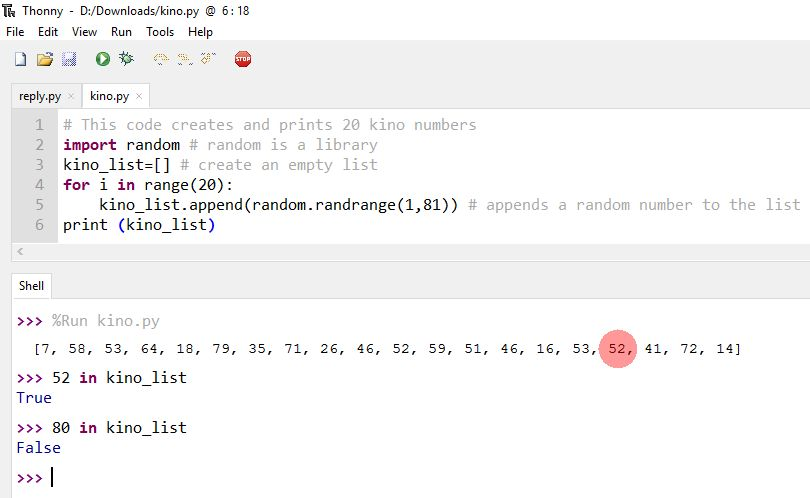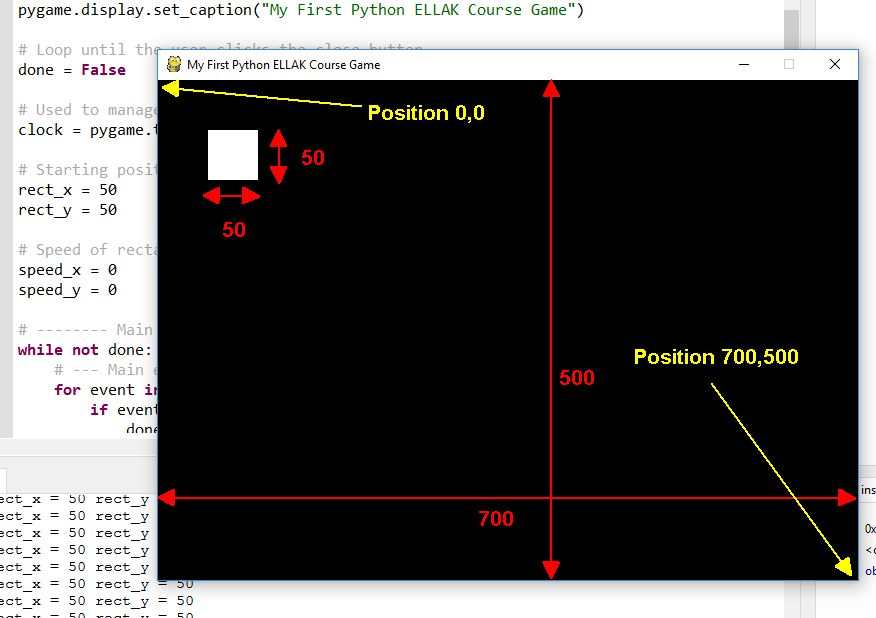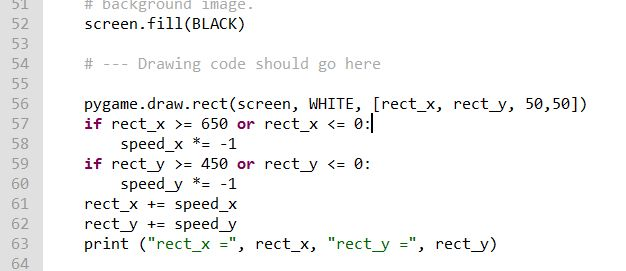# Week 3 - Section 2 - Graphics

 Site: ΕΛ/ΛΑΚ Moodle Course: Python Lab - May 2017 Book: Week 3 - Section 2 - Graphics
 Printed by: Guest user Date: Monday, 2 October 2023, 3:20 PM

## Description

In this section we will learn about Graphics

## Chapter 5.1 - What is in a list?

When we created a list of 20 different numbers (kino) in previous lesson (Chapter 4.4) we asked you if the numbers in the list were unique. The answer is NO because when we add random numbers we can add more than once the same number.

So if you have saved the kino program open it or else go to chapter 4.4,  copy the code from there and save it as kino.

After executing the code look at the output find a number and write the commands as in the picture.With in command we can search inside a list.

Copy and paste the following code to Thonny and save it as kino2 :

# This code creates and prints 20 Unique kino numbers import random # random is a library kino_list=[] # create an empty list for i in range(20): new_number = random.randrange(1,81) while new_number in kino_list: # if new number is in the list select another until new_number = random.randrange(1,81) # it is not in the list kino_list.append(random.randrange(1,81)) # appends a random number to the list print (kino_list)

Compare it with the previous code of kino program. Are there 20 unique numbers now?

## Chapter 5.2 - List Methods

5.2 - List Methods

Following there are most of the list methods.

>>> fruits = ['orange', 'apple', 'pear', 'banana', 'kiwi', 'apple', 'banana']

>>> fruits.count('apple')

2

>>> fruits.count('tangerine')

0

>>> fruits.index('banana')

3

>>> fruits.index('banana', 4)  # Find next banana starting a position 4

6

>>> fruits.reverse()

>>> fruits

['banana', 'apple', 'kiwi', 'banana', 'pear', 'apple', 'orange']

>>> fruits.append('grape')

>>> fruits

['banana', 'apple', 'kiwi', 'banana', 'pear', 'apple', 'orange', 'grape']

>>> fruits.sort()

>>> fruits

['apple', 'apple', 'banana', 'banana', 'grape', 'kiwi', 'orange', 'pear']

>>> fruits.pop()

'pear'

## Chapter 5.3 - Graphics

5.3 - Graphics

Open the Basic Pygame Template file (click here if you haven't saved it).

If you can't remember what is the purpose of every command go back to Chapter 4.8 and review.

Around line 43 there is a command to fill the background with WHITE color. Change this line so the background is BLACK and run the code to see the difference. (you should change the line to  screen.fill(BLACK) )

Now between lines 45 and 47 we will write code to create a WHITE rectangle. In line 46 write
pygame.draw.rect(screen, WHITE, [50, 50, 50, 50])

Pygame is the library, draw is a method, rect (for rectangle) is a type, screen is where to draw the first two numbers is the top left coordinates and the second two numbers are the width and height. You can experiment changing these numbers (consider that your screen is 700 x 500) and the color to one of the previously defined.You can view what other shapes you can draw here

Try to draw another shape. This time draw a circle

If you didn't succeed try the following line :

pygame.draw.circle(screen, RED, [200, 140], 30)

[100, 80] is the center of the circle and 30 its radious.

Change the code adding another number before closing the parenthesis like this :

pygame.draw.rect(screen, WHITE, [50, 50, 50, 50], 7)
pygame.draw.circle(screen, RED, [200, 140], 30, 5)

For now comment the line which draws the circle (use a # in front of the line) and leave the rectangle fully filled.

pygame.draw.rect(screen, WHITE, [50, 50, 50, 50])
# pygame.draw.circle(screen, RED, [200, 140], 30, 5)

## Chapter 5.4 - Move on

5.4 - Move on

This screen that we have created is updated 60 times per second with the command clock.tick(60) but because we don't change anything it is the same picture 60 times a second.

Now we are going to move the rectangle. How can we do this? Which of the for numbers [50, 50, 50, 50] is the position of the rectangle? If you don't remember go to the previous page and look at the picture.

We are going to replace the numbers with variables. Replace the draw command with these eight lines.

rect_x = 50    # X starting position of rectangle
rect_y = 50    # Y starting position of rectangle
speed_x = 5   # X speed of rectangle
speed_y = 5   # Y speed of rectangle
pygame.draw.rect(screen, WHITE, [rect_x, rect_y, 50,50])
rect_x += speed_x
rect_y += speed_y
print ("rect_x =", rect_x, "rect_y =", rect_y)

Nothing is happening. Why? Because 60 times per second rect_x and rect_y become 50 and then 55.

What should we do? Put the first four lines outside the loop. Cut and paste them before line 28 (#Main program loop) and don't forget the indentation.

Wow!! A moving rectangle!!

## Chapter 5.5 - And stay on

5.5 - And stay on

So we managed to make the rectangle to move. Now we are going to control its movement. Download the code from here and open it to Thonny.

Change lines 33 and 34 using different integer numbers (0, 1, 2,...) and every time execute the code. Try with negative numbers (ex -5 or -1).

What happens if speed_x or speed_y is 0? What happens if they are negative?

speed = 0 means that rectangle is not moving
speed > 0 means that rectangle moves to one direction
speed < 0 means that rectangle moves to the other direction

How can we change the direction? Try this in CLI :

>>> number = 5
>>> print (number)
>>> number *= -1
>>> print(number)We will add this lines of code to the loop after drawing the rectangle (after line 56) and move the other lines downwards :

 if rect_x >= 650 or rect_x <= 0: speed_x *= -1 if rect_y >= 450 or rect_y <= 0: speed_y *= -1